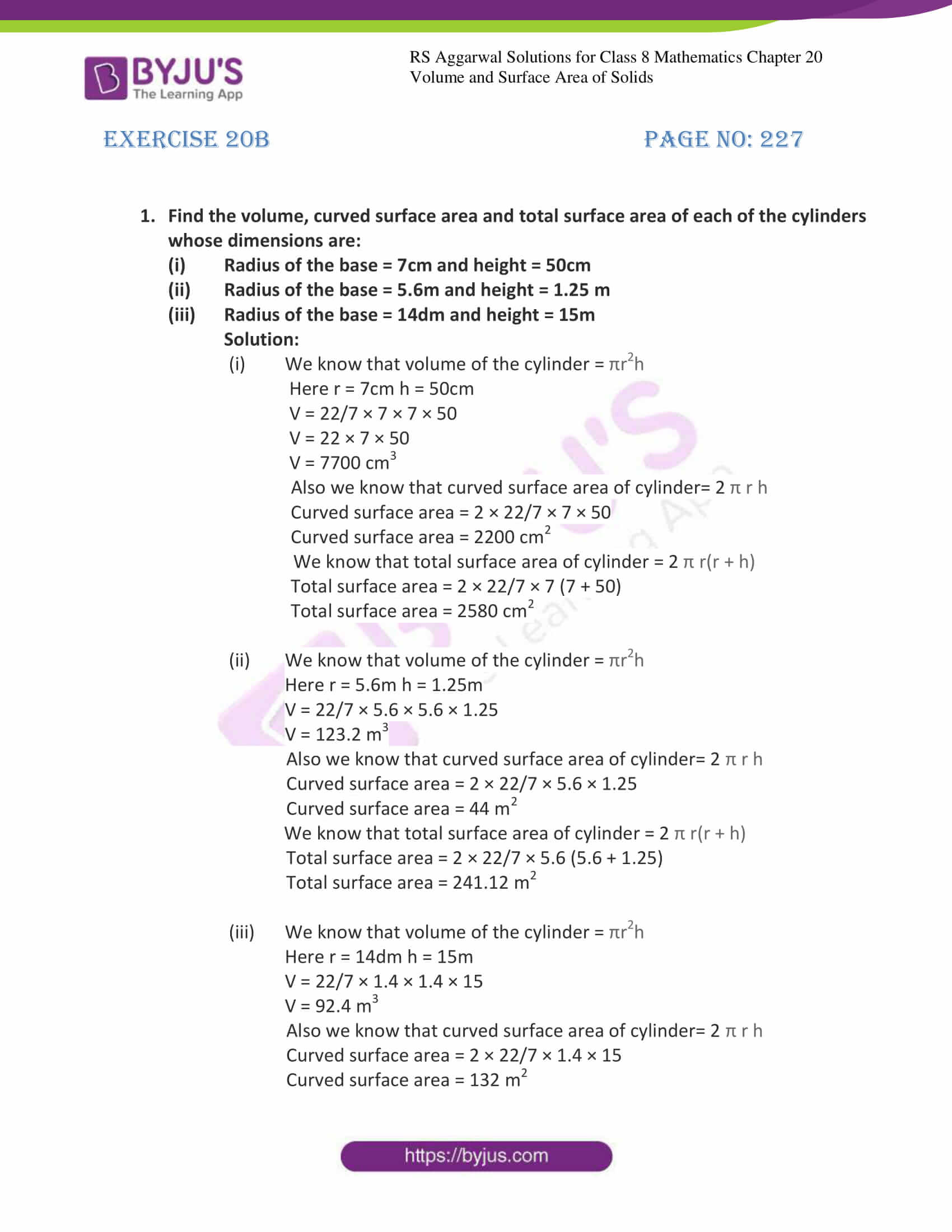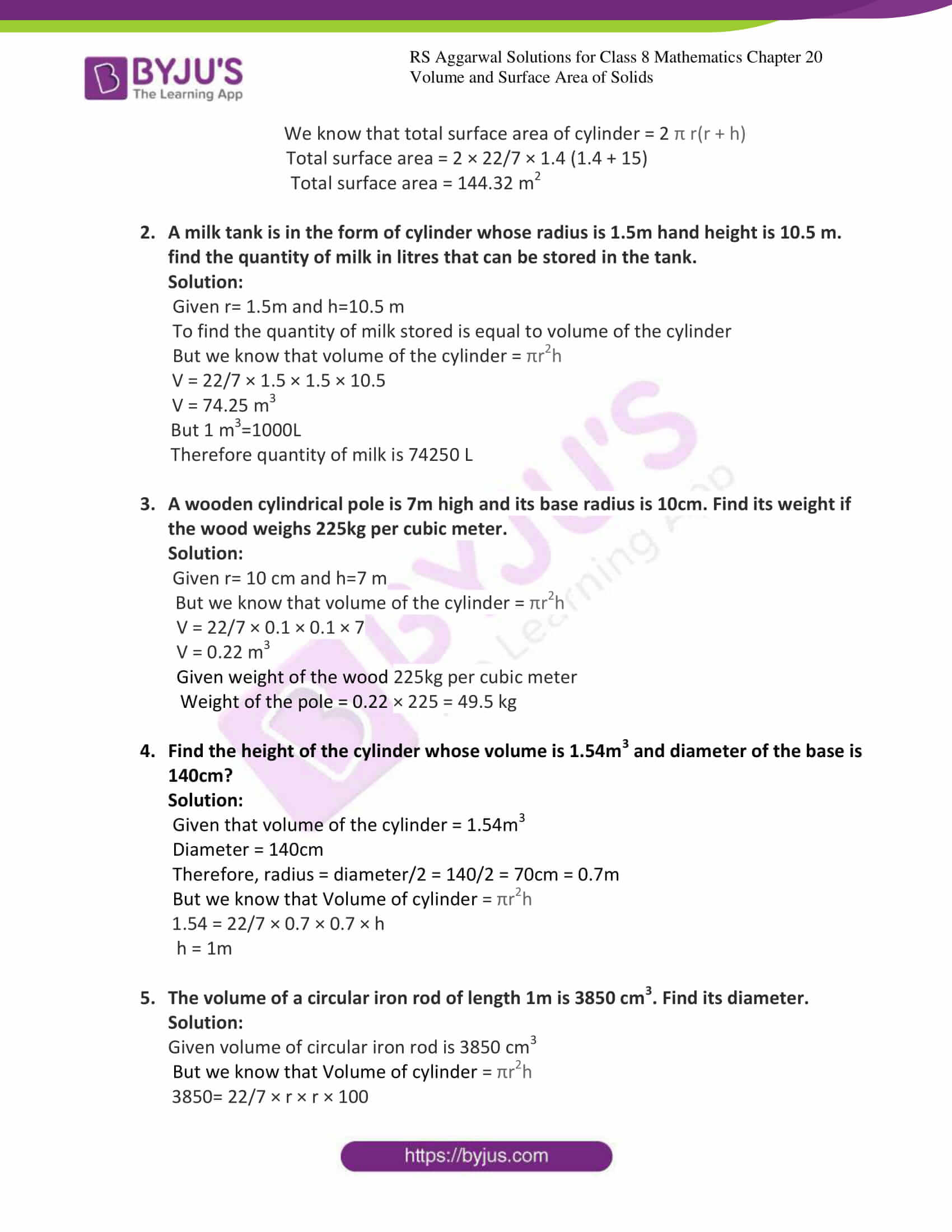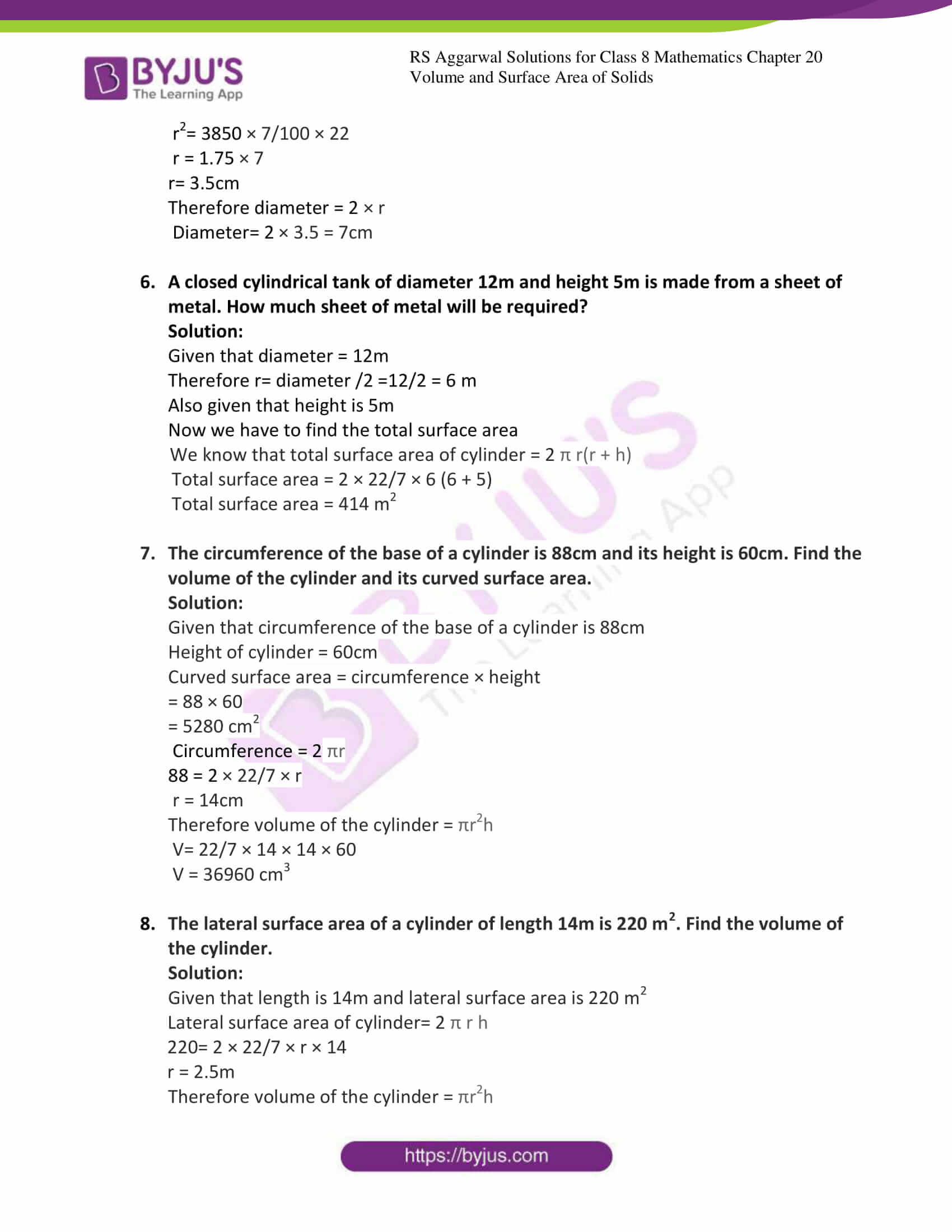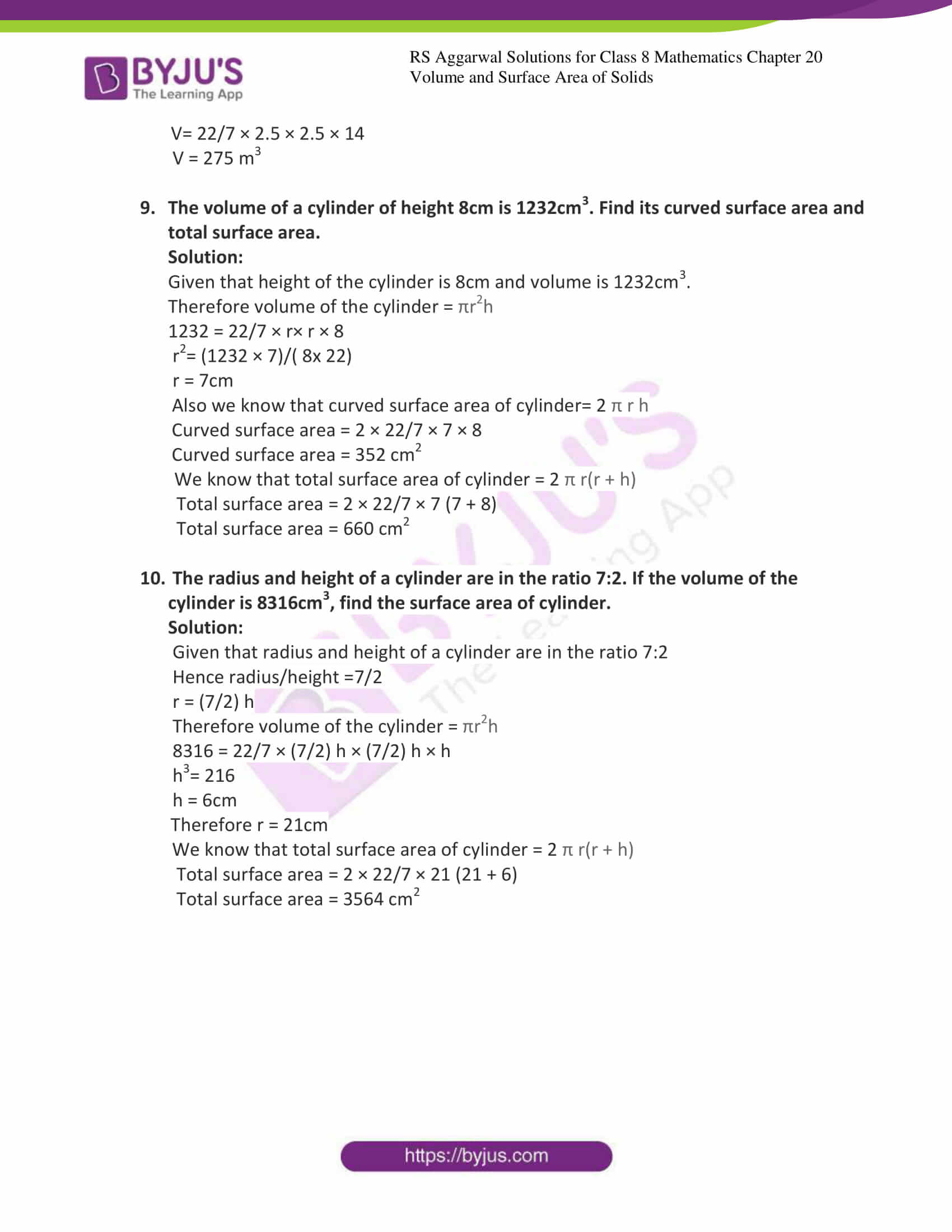# RS Aggarwal Solutions for Class 8 Maths Chapter 20 - Volume and Surface Area of Solids Exercise 20B

Learners can download the RS Aggarwal Solutions for the Exercise 20B of Class 8 Maths Chapter 20, Volume and Surface Area of Solids from the link available here. The questions present in this exercise has solved by the BYJU’S expert team. This exercise contains twenty-one questions. By practising the RS Aggarwal Solutions for Class 8, students will be able to grasp the concepts perfectly. Those who aim to score high in the Maths of Class 8 are advised to practice all the questions present in RS Aggarwal as many times as possible.

## Download PDF of RS Aggarwal Solutions for Class 8 Maths Chapter 20 – Volume And Surface Area Of Solids – Exercise 20B### Access answers to Maths RS Aggarwal Solutions for Class 8 Chapter 20 – Volume and Surface Area of Solids Exercise 20B

Exercise 20B Page No: 227

1. Find the volume, curved surface area and total surface area of each of the cylinders whose dimensions are:

(i) Radius of the base = 7cm and height = 50cm

(ii) Radius of the base = 5.6m and height = 1.25 m

(iii) Radius of the base = 14dm and height = 15m

Solution:

(i) We know that volume of the cylinder = πr2h

Here r = 7cm h = 50cm

V = 22/7 × 7 × 7 × 50

V = 22 × 7 × 50

V = 7700 cm3

Also we know that curved surface area of cylinder= 2 π r h

Curved surface area = 2 × 22/7 × 7 × 50

Curved surface area = 2200 cm2

We know that total surface area of cylinder = 2 π r(r + h)

Total surface area = 2 × 22/7 × 7 (7 + 50)

Total surface area = 2580 cm2

(ii) We know that volume of the cylinder = πr2h

Here r = 5.6m h = 1.25m

V = 22/7 × 5.6 × 5.6 × 1.25

V = 123.2 m3

Also we know that curved surface area of cylinder= 2 π r h

Curved surface area = 2 × 22/7 × 5.6 × 1.25

Curved surface area = 44 m2

We know that total surface area of cylinder = 2 π r(r + h)

Total surface area = 2 × 22/7 × 5.6 (5.6 + 1.25)

Total surface area = 241.12 m2

(iii) We know that volume of the cylinder = πr2h

Here r = 14dm h = 15m

V = 22/7 × 1.4 × 1.4 × 15

V = 92.4 m3

Also we know that curved surface area of cylinder= 2 π r h

Curved surface area = 2 × 22/7 × 1.4 × 15

Curved surface area = 132 m2

We know that total surface area of cylinder = 2 π r(r + h)

Total surface area = 2 × 22/7 × 1.4 (1.4 + 15)

Total surface area = 144.32 m2

2. A milk tank is in the form of cylinder whose radius is 1.5m hand height is 10.5 m. find the quantity of milk in litres that can be stored in the tank.

Solution:

Given r= 1.5m and h=10.5 m

To find the quantity of milk stored is equal to volume of the cylinder

But we know that volume of the cylinder = πr2h

V = 22/7 × 1.5 × 1.5 × 10.5

V = 74.25 m3

But 1 m3=1000L

Therefore quantity of milk is 74250 L

3. A wooden cylindrical pole is 7m high and its base radius is 10cm. Find its weight if the wood weighs 225kg per cubic meter.

Solution:

Given r= 10 cm and h=7 m

But we know that volume of the cylinder = πr2h

V = 22/7 × 0.1 × 0.1 × 7

V = 0.22 m3

Given weight of the wood 225kg per cubic meter

Weight of the pole = 0.22 × 225 = 49.5 kg

4. Find the height of the cylinder whose volume is 1.54m3 and diameter of the base is 140cm?

Solution:

Given that volume of the cylinder = 1.54m3

Diameter = 140cm

Therefore, radius = diameter/2 = 140/2 = 70cm = 0.7m

But we know that Volume of cylinder = πr2h

1.54 = 22/7 × 0.7 × 0.7 × h

h = 1m

5. The volume of a circular iron rod of length 1m is 3850 cm3. Find its diameter.

Solution:

Given volume of circular iron rod is 3850 cm3

But we know that Volume of cylinder = πr2h

3850= 22/7 × r × r × 100

r2= 3850 × 7/100 × 22

r = 1.75 × 7

r= 3.5cm

Therefore diameter = 2 × r

Diameter= 2 × 3.5 = 7cm

6. A closed cylindrical tank of diameter 12m and height 5m is made from a sheet of metal. How much sheet of metal will be required?

Solution:

Given that diameter = 12m

Therefore r= diameter /2 =12/2 = 6 m

Also given that height is 5m

Now we have to find the total surface area

We know that total surface area of cylinder = 2 π r(r + h)

Total surface area = 2 × 22/7 × 6 (6 + 5)

Total surface area = 414 m2

7. The circumference of the base of a cylinder is 88cm and its height is 60cm. Find the volume of the cylinder and its curved surface area.

Solution:

Given that circumference of the base of a cylinder is 88cm

Height of cylinder = 60cm

Curved surface area = circumference × height

= 88 × 60

= 5280 cm2

Circumference = 2 πr

88 = 2 × 22/7 × r

r = 14cm

Therefore volume of the cylinder = πr2h

V= 22/7 × 14 × 14 × 60

V = 36960 cm3

8. The lateral surface area of a cylinder of length 14m is 220 m2. Find the volume of the cylinder.

Solution:

Given that length is 14m and lateral surface area is 220 m2

Lateral surface area of cylinder= 2 π r h

220= 2 × 22/7 × r × 14

r = 2.5m

Therefore volume of the cylinder = πr2h

V= 22/7 × 2.5 × 2.5 × 14

V = 275 m3

9. The volume of a cylinder of height 8cm is 1232cm3. Find its curved surface area and total surface area.

Solution:

Given that height of the cylinder is 8cm and volume is 1232cm3.

Therefore volume of the cylinder = πr2h

1232 = 22/7 × r× r × 8

r2= (1232 × 7)/( 8x 22)

r = 7cm

Also we know that curved surface area of cylinder= 2 π r h

Curved surface area = 2 × 22/7 × 7 × 8

Curved surface area = 352 cm2

We know that total surface area of cylinder = 2 π r(r + h)

Total surface area = 2 × 22/7 × 7 (7 + 8)

Total surface area = 660 cm2

10. The radius and height of a cylinder are in the ratio 7:2. If the volume of the cylinder is 8316cm3, find the surface area of cylinder.

Solution:

Given that radius and height of a cylinder are in the ratio 7:2

r = (7/2) h

Therefore volume of the cylinder = πr2h

8316 = 22/7 × (7/2) h × (7/2) h × h

h3= 216

h = 6cm

Therefore r = 21cm

We know that total surface area of cylinder = 2 π r(r + h)

Total surface area = 2 × 22/7 × 21 (21 + 6)

Total surface area = 3564 cm2

## RS Aggarwal Solutions for Class 8 Maths Chapter 20 – Volume and Surface Area of Solids Exercise 20B

Exercise 20B of RS Aggarwal Solutions for Class 8 Maths Chapter 20 Volume and Surface area of Solids deals with the basic concepts related to the total Surface Area of Solids. We can say that this exercise mainly deals with the formulae to calculate the Volume and Surface Area of Solids that the students have learned in this Chapter. Some of the topics focused prior to exercise 20B include the following.

• Formulae for Volume and Surface Area of a cuboid
• Formulae for Volume and Surface Area of a cube
• Formulae for Volume and Surface Area of a cylinder

The RS Aggarwal Solutions can help the students in practising and learning each and every concept as it provides solutions to all questions asked in the RS Aggarwal textbook.